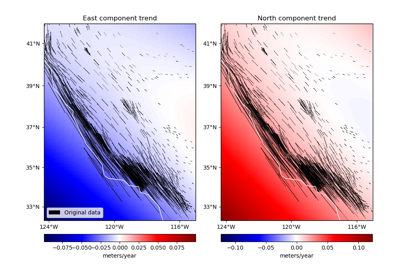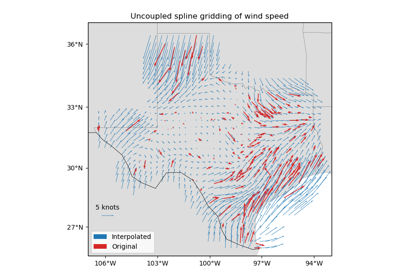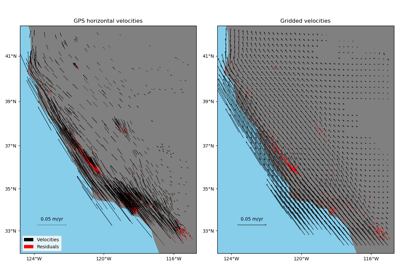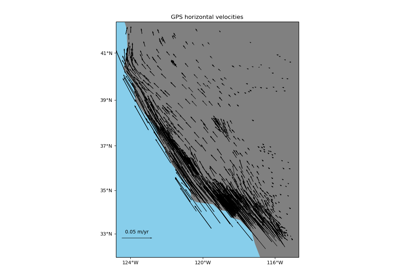# verde.Vector¶

class verde.Vector(components)[source]

Fit an estimator to each component of multi-component vector data.

Provides a convenient way of fitting and gridding vector data using scalar gridders and estimators.

Each data component provided to fit is fitted to a separated estimator. Methods like grid and predict will operate on the multiple components simultaneously.

Warning

Never pass code like this as input to this class: [vd.Trend(1)]*3. This creates 3 references to the same instance of Trend, which means that they will all get the same coefficients after fitting. Use a list comprehension instead: [vd.Trend(1) for i in range(3)].

Parameters: components : tuple or list A tuple or list of the estimator/gridder instances used for each component. The estimators will be applied for each data component in the same order that they are given here.

verde.Chain
Chain filtering operations to fit on each subsequent output.
Attributes: components : tuple Tuple of the fitted estimators on each component of the data. region_ : tuple The boundaries ([W, E, S, N]) of the data used to fit the interpolator. Used as the default region for the grid and scatter methods.

Methods

 filter(coordinates, data[, weights]) Filter the data through the gridder and produce residuals. fit(coordinates, data[, weights]) Fit the estimators to the given multi-component data. get_params([deep]) Get parameters for this estimator. grid([region, shape, spacing, dims, …]) Interpolate the data onto a regular grid. predict(coordinates) Evaluate each data component on a set of points. profile(point1, point2, size[, dims, …]) Interpolate data along a profile between two points. scatter([region, size, random_state, dims, …]) Interpolate values onto a random scatter of points. score(coordinates, data[, weights]) Score the gridder predictions against the given data. set_params(**params) Set the parameters of this estimator.

## Examples using verde.Vector¶Trends in vector dataGridding 2D vectors (uncoupled)Gridding 2D vectors (coupled)Vector Data

Vector.filter(coordinates, data, weights=None)

Filter the data through the gridder and produce residuals.

Calls fit on the data, evaluates the residuals (data - predicted data), and returns the coordinates, residuals, and weights.

No very useful by itself but this interface makes gridders compatible with other processing operations and is used by verde.Chain to join them together (for example, so you can fit a spline on the residuals of a trend).

Parameters: coordinates : tuple of arrays Arrays with the coordinates of each data point. Should be in the following order: (easting, northing, vertical, …). data : array or tuple of arrays The data values of each data point. If the data has more than one component, data must be a tuple of arrays (one for each component). weights : None or array or tuple of arrays If not None, then the weights assigned to each data point. If more than one data component is provided, you must provide a weights array for each data component (if not None). coordinates, residuals, weights The coordinates and weights are same as the input. Residuals are the input data minus the predicted data.
Vector.fit(coordinates, data, weights=None)[source]

Fit the estimators to the given multi-component data.

The data region is captured and used as default for the grid and scatter methods.

All input arrays must have the same shape. If weights are given, there must be a separate array for each component of the data.

Parameters: coordinates : tuple of arrays Arrays with the coordinates of each data point. Should be in the following order: (easting, northing, vertical, …). Only easting and northing will be used, all subsequent coordinates will be ignored. data : tuple of array The data values of each component at each data point. Must be a tuple. weights : None or tuple of array If not None, then the weights assigned to each data point of each data component. Typically, this should be 1 over the data uncertainty squared. self Returns this estimator instance for chaining operations.
Vector.get_params(deep=True)

Get parameters for this estimator.

Parameters: deep : boolean, optional If True, will return the parameters for this estimator and contained subobjects that are estimators. params : mapping of string to any Parameter names mapped to their values.
Vector.grid(region=None, shape=None, spacing=None, dims=None, data_names=None, projection=None, **kwargs)

Interpolate the data onto a regular grid.

The grid can be specified by either the number of points in each dimension (the shape) or by the grid node spacing. See verde.grid_coordinates for details. Other arguments for verde.grid_coordinates can be passed as extra keyword arguments (kwargs) to this method.

If the interpolator collected the input data region, then it will be used if region=None. Otherwise, you must specify the grid region.

Use the dims and data_names arguments to set custom names for the dimensions and the data field(s) in the output xarray.Dataset. Default names will be provided if none are given.

Parameters: region : list = [W, E, S, N] The boundaries of a given region in Cartesian or geographic coordinates. shape : tuple = (n_north, n_east) or None The number of points in the South-North and West-East directions, respectively. spacing : tuple = (s_north, s_east) or None The grid spacing in the South-North and West-East directions, respectively. dims : list or None The names of the northing and easting data dimensions, respectively, in the output grid. Defaults to ['northing', 'easting']. NOTE: This is an exception to the “easting” then “northing” pattern but is required for compatibility with xarray. data_names : list of None The name(s) of the data variables in the output grid. Defaults to ['scalars'] for scalar data, ['east_component', 'north_component'] for 2D vector data, and ['east_component', 'north_component', 'vertical_component'] for 3D vector data. projection : callable or None If not None, then should be a callable object projection(easting, northing) -> (proj_easting, proj_northing) that takes in easting and northing coordinate arrays and returns projected northing and easting coordinate arrays. This function will be used to project the generated grid coordinates before passing them into predict. For example, you can use this to generate a geographic grid from a Cartesian gridder. grid : xarray.Dataset The interpolated grid. Metadata about the interpolator is written to the attrs attribute.

verde.grid_coordinates
Generate the coordinate values for the grid.
Vector.predict(coordinates)[source]

Evaluate each data component on a set of points.

Requires a fitted estimator (see fit).

Parameters: coordinates : tuple of arrays Arrays with the coordinates of each data point. Should be in the following order: (easting, northing, vertical, …). Only easting and northing will be used, all subsequent coordinates will be ignored. data : tuple of array The values for each vector component evaluated on the given points. The order of components will be the same as was provided to fit.
Vector.profile(point1, point2, size, dims=None, data_names=None, projection=None, **kwargs)

Interpolate data along a profile between two points.

Generates the profile along a straight line assuming Cartesian distances. Point coordinates are generated by verde.profile_coordinates. Other arguments for this function can be passed as extra keyword arguments (kwargs) to this method.

Use the dims and data_names arguments to set custom names for the dimensions and the data field(s) in the output pandas.DataFrame. Default names are provided.

Includes the calculated Cartesian distance from point1 for each data point in the profile.

Parameters: point1 : tuple The easting and northing coordinates, respectively, of the first point. point2 : tuple The easting and northing coordinates, respectively, of the second point. size : int The number of points to generate. dims : list or None The names of the northing and easting data dimensions, respectively, in the output dataframe. Defaults to ['northing', 'easting']. NOTE: This is an exception to the “easting” then “northing” pattern but is required for compatibility with xarray. data_names : list of None The name(s) of the data variables in the output dataframe. Defaults to ['scalars'] for scalar data, ['east_component', 'north_component'] for 2D vector data, and ['east_component', 'north_component', 'vertical_component'] for 3D vector data. projection : callable or None If not None, then should be a callable object projection(easting, northing) -> (proj_easting, proj_northing) that takes in easting and northing coordinate arrays and returns projected northing and easting coordinate arrays. This function will be used to project the generated profile coordinates before passing them into predict. For example, you can use this to generate a geographic profile from a Cartesian gridder. table : pandas.DataFrame The interpolated values along the profile.
Vector.scatter(region=None, size=300, random_state=0, dims=None, data_names=None, projection=None, **kwargs)

Interpolate values onto a random scatter of points.

Point coordinates are generated by verde.scatter_points. Other arguments for this function can be passed as extra keyword arguments (kwargs) to this method.

If the interpolator collected the input data region, then it will be used if region=None. Otherwise, you must specify the grid region.

Use the dims and data_names arguments to set custom names for the dimensions and the data field(s) in the output pandas.DataFrame. Default names are provided.

Parameters: region : list = [W, E, S, N] The boundaries of a given region in Cartesian or geographic coordinates. size : int The number of points to generate. random_state : numpy.random.RandomState or an int seed A random number generator used to define the state of the random permutations. Use a fixed seed to make sure computations are reproducible. Use None to choose a seed automatically (resulting in different numbers with each run). dims : list or None The names of the northing and easting data dimensions, respectively, in the output dataframe. Defaults to ['northing', 'easting']. NOTE: This is an exception to the “easting” then “northing” pattern but is required for compatibility with xarray. data_names : list of None The name(s) of the data variables in the output dataframe. Defaults to ['scalars'] for scalar data, ['east_component', 'north_component'] for 2D vector data, and ['east_component', 'north_component', 'vertical_component'] for 3D vector data. projection : callable or None If not None, then should be a callable object projection(easting, northing) -> (proj_easting, proj_northing) that takes in easting and northing coordinate arrays and returns projected northing and easting coordinate arrays. This function will be used to project the generated scatter coordinates before passing them into predict. For example, you can use this to generate a geographic scatter from a Cartesian gridder. table : pandas.DataFrame The interpolated values on a random set of points.
Vector.score(coordinates, data, weights=None)

Score the gridder predictions against the given data.

Calculates the R^2 coefficient of determination of between the predicted values and the given data values. A maximum score of 1 means a perfect fit. The score can be negative.

If the data has more than 1 component, the scores of each component will be averaged.

Parameters: coordinates : tuple of arrays Arrays with the coordinates of each data point. Should be in the following order: (easting, northing, vertical, …). data : array or tuple of arrays The data values of each data point. If the data has more than one component, data must be a tuple of arrays (one for each component). weights : None or array or tuple of arrays If not None, then the weights assigned to each data point. If more than one data component is provided, you must provide a weights array for each data component (if not None). score : float The R^2 score
Vector.set_params(**params)

Set the parameters of this estimator.

The method works on simple estimators as well as on nested objects (such as pipelines). The latter have parameters of the form <component>__<parameter> so that it’s possible to update each component of a nested object.

Returns: self